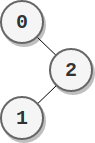Formatted question description: https://leetcode.ca/all/2049.html

# 2049. Count Nodes With the Highest Score (Medium)

There is a binary tree rooted at 0 consisting of n nodes. The nodes are labeled from 0 to n - 1. You are given a 0-indexed integer array parents representing the tree, where parents[i] is the parent of node i. Since node 0 is the root, parents == -1.

Each node has a score. To find the score of a node, consider if the node and the edges connected to it were removed. The tree would become one or more non-empty subtrees. The size of a subtree is the number of the nodes in it. The score of the node is the product of the sizes of all those subtrees.

Return the number of nodes that have the highest score.

Example 1:Input: parents = [-1,2,0,2,0]
Output: 3
Explanation:
- The score of node 0 is: 3 * 1 = 3
- The score of node 1 is: 4 = 4
- The score of node 2 is: 1 * 1 * 2 = 2
- The score of node 3 is: 4 = 4
- The score of node 4 is: 4 = 4
The highest score is 4, and three nodes (node 1, node 3, and node 4) have the highest score.


Example 2:Input: parents = [-1,2,0]
Output: 2
Explanation:
- The score of node 0 is: 2 = 2
- The score of node 1 is: 2 = 2
- The score of node 2 is: 1 * 1 = 1
The highest score is 2, and two nodes (node 0 and node 1) have the highest score.


Constraints:

• n == parents.length
• 2 <= n <= 105
• parents == -1
• 0 <= parents[i] <= n - 1 for i != 0
• parents represents a valid binary tree.

Similar Questions:

## Solution 1. Post-order Traversal

Post-order traverse the tree. For each node, calculate its score by multiplying the node count of its left subtree, right subtree and nodes not in the current subtree (ignoring 0). Count the nodes with the maximum score.

// OJ: https://leetcode.com/problems/count-nodes-with-the-highest-score/
// Time: O(N)
// Space: O(N)
class Solution {
public:
int countHighestScoreNodes(vector<int>& P) {
long N = P.size(), ans = 0, maxScore = 0;
vector<vector<int>> G(N); // build the graph -- G[i] is a list of the children of node i.
for (int i = 1; i < N; ++i) G[P[i]].push_back(i);
function<int(int)> dfs = [&](int u) { // Post-order traversal. Returns the size of the subtree rooted at node u.
long score = 1, cnt = 1;
for (int v : G[u]) {
int c = dfs(v);
cnt += c;
score *= c;
}
long other = N - cnt; // The count of nodes not in this subtree rooted at node u.
if (other) score *= other;
if (score > maxScore) {
maxScore = score;
ans = 1;
} else if (score == maxScore) ++ans;
return cnt;
};
dfs(0);
return ans;
}
};


## Discuss

https://leetcode.com/problems/count-nodes-with-the-highest-score/discuss/1537494/C%2B%2B-Post-order-Traversal